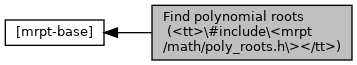Main MRPT website > C++ reference for MRPT 1.5.7
Find polynomial roots (`#include <mrpt/math/poly_roots.h>`)

## Detailed Description

Collaboration diagram for Find polynomial roots (`#include <mrpt/math/poly_roots.h>`):## Functions

int BASE_IMPEXP mrpt::math::solve_poly3 (double *x, double a, double b, double c) MRPT_NO_THROWS
Solves cubic equation `x^3 + a*x^2 + b*x + c = 0`. More...

int BASE_IMPEXP mrpt::math::solve_poly4 (double *x, double a, double b, double c, double d) MRPT_NO_THROWS
Solves quartic equation `x^4 + a*x^3 + b*x^2 + c*x + d = 0` by Dekart-Euler method. More...

int BASE_IMPEXP mrpt::math::solve_poly5 (double *x, double a, double b, double c, double d, double e) MRPT_NO_THROWS
Solves equation `x^5 + a*x^4 + b*x^3 + c*x^2 + d*x + e = 0`. More...

int BASE_IMPEXP mrpt::math::solve_poly4Bi (double *x, double b, double d) MRPT_NO_THROWS
Solve equation x^4 + b*x^2 + d = 0. More...

int BASE_IMPEXP mrpt::math::solve_poly4De (double *x, double b, double c, double d) MRPT_NO_THROWS
Solve equation x^4 + b*x^2 + c*x + d = 0. More...

int BASE_IMPEXP mrpt::math::solve_poly2 (double a, double b, double c, double &r1, double &r2) MRPT_NO_THROWS
Solves equation `a*x^2 + b*x + c = 0`. More...

## ◆ solve_poly2()

 int mrpt::math::solve_poly2 ( double a, double b, double c, double & r1, double & r2 )

Solves equation `a*x^2 + b*x + c = 0`.

Returns the number of real roots: either 0 or 2; or 1 if a=0 (in this case the root is in r1). r1, r2 are the roots. (r1<=r2)

Note
Based on `poly34.h`, by Khashin S.I. http://math.ivanovo.ac.ru/dalgebra/Khashin/index.html - khash2 (at) gmail.com

Definition at line 302 of file poly_roots.cpp.

References eps, and SWAP.

## ◆ solve_poly3()

 int mrpt::math::solve_poly3 ( double * x, double a, double b, double c )

Solves cubic equation `x^3 + a*x^2 + b*x + c = 0`.

Returns the number of real roots `N`<=3. The roots are returned in the first entries of `x`, i.e. `x` if N=1, `x` and `x` if N=2, etc.

Parameters
 x array of size 3
Note
Based on `poly34.h`, by Khashin S.I. http://math.ivanovo.ac.ru/dalgebra/Khashin/index.html - khash2 (at) gmail.com

Definition at line 29 of file poly_roots.cpp.

References mrpt::obs::gnss::a2, eps, and TwoPi.

Referenced by SolveP4De(), TEST(), and mrpt::nav::CPTG_Holo_Blend::updateTPObstacleSingle().

## ◆ solve_poly4()

 int mrpt::math::solve_poly4 ( double * x, double a, double b, double c, double d )

Solves quartic equation `x^4 + a*x^3 + b*x^2 + c*x + d = 0` by Dekart-Euler method.

Returns the number of real roots `N`<=4:

• return 4: 4 real roots x, x, x, x, possible multiple roots
• return 2: 2 real roots x, x and complex x+-i*x,
• return 0: two pair of complex roots: x+-i*x, x+-i*x,

The roots are returned in the first entries of `x`, i.e. `x` if N=1, `x` and `x` if N=2, etc.

Parameters
 x array of size 4
Note
Based on `poly34.h`, by Khashin S.I. http://math.ivanovo.ac.ru/dalgebra/Khashin/index.html - khash2 (at) gmail.com

Definition at line 215 of file poly_roots.cpp.

References mrpt::obs::gnss::b1, N4Step(), and SolveP4De().

## ◆ solve_poly4Bi()

 int BASE_IMPEXP mrpt::math::solve_poly4Bi ( double * x, double b, double d )

Solve equation x^4 + b*x^2 + d = 0.

## ◆ solve_poly4De()

 int BASE_IMPEXP mrpt::math::solve_poly4De ( double * x, double b, double c, double d )

Solve equation x^4 + b*x^2 + c*x + d = 0.

## ◆ solve_poly5()

 int mrpt::math::solve_poly5 ( double * x, double a, double b, double c, double d, double e )

Solves equation `x^5 + a*x^4 + b*x^3 + c*x^2 + d*x + e = 0`.

Returns the number of real roots `N`<=5. The roots are returned in the first entries of `x`, i.e. `x` if N=1, `x` and `x` if N=2, etc.

Parameters
 x array of size 5
Note
Based on `poly34.h`, by Khashin S.I. http://math.ivanovo.ac.ru/dalgebra/Khashin/index.html - khash2 (at) gmail.com

Definition at line 293 of file poly_roots.cpp.

 Page generated by Doxygen 1.8.14 for MRPT 1.5.7 Git: 8277875f6 Mon Jun 11 02:47:32 2018 +0200 at lun oct 28 01:50:49 CET 2019